# Programming - Java Graph (Backtracking) Hamiltonian Circuit Algorithm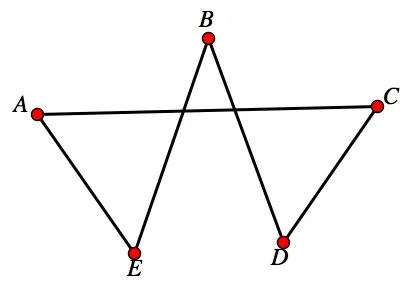Hello its me again Drifter Programming. Today we get back into Java Graph Algorithms to talk about how we find a Hamiltonian Circuit inside of a Graph. I will first talk about what this problem is all about, the naive algorithm steps and lastly get into an Backtracking algorithm that is much faster and that we will also implement in Java. So, without further do, let's get started!

## Hamiltonian Circuit Problem:

A Hamiltonian or traceable path inside of an undirected or directed Graph is a path where each Vertex gets visited only one time. If we also return to the starting Vertex and create a circle, then we are talking about a Hamiltonian Circuit. The complexity of this problem is rated as NP-Complete, which means that the solutions can be verified in polynomial time. This also means that we will need a lot of steps to get the best solution and sometimes their might even exist infinitely more better solutions! In this problem for example we can have N! combinations of those N Vertices to form a Circuit (or Path) and so we have a factorial complexity that is pretty bad!

So, what do we need?

Well, we need an Algorithm that takes in a Graph and gives us a Hamiltonian Circuit Solution as the result. If we want to get the best possible solution or find out all the possible solutions/circuits, then we will have to take the Naive approach and check each and every combination. But, if we just want to know if a circuit exists then we can use the Naive approach and stop when a circuit exists or we can use the Backtracking algorithm that I will get into later on...

## Naive Algorithm Pseudocode:

The naive algorithm generates all possible combinations of vertices and prints out those that are a solution. We could also stop when we find a solution, but either way the worst case senario would be to check all the n! combinations.

So, the naive algorithm has the following Pseudocode:

``````while there are untried combinations
{
generate the next combination
if ( there are edges between two consecutive vertices of this
combination and there is an edge from the last vertex to
the first ).
{
print this combination;
break; // if we only want to find one (or that one exists), else delete this
}
}``````

## Backtracking Algorithm in Java:

The backtracking algorithm creates an empty path array and adds vertex 0 to it. Then it adds other vertices, starting from vertex 1 and checks if this vertex can be added (not adjacent to a previously added vertex and also not yet added). If it finds such a vertex then it adds the vertex to the path/circuit. It does so recursively/continuously, going back (backtracking) when the selection of some vertex gave no solution, trying out other combinations until none remain or a solution was found. Using this principle we mostly minimize the steps needed, cause whole subtrees of combinations can be left out!

To implement this algorithm in Java I will use a edited variant of my classic Adjacency Matrix Implementation, where 0 will mean Edge does not exist and 1 that the Edge exists. The whole algorithm/function will consist of 3 functions. The first will check if the vertex can be added. The second one will recursively add vertices, backtracking if needed and returning if a circuit was found or not. The last one (highest level one) calls the recursive one and prints out if a solution was found or not, and prints the path/circuit if one was found!

Let's now get into the Java Code...

The Graph class looks like this: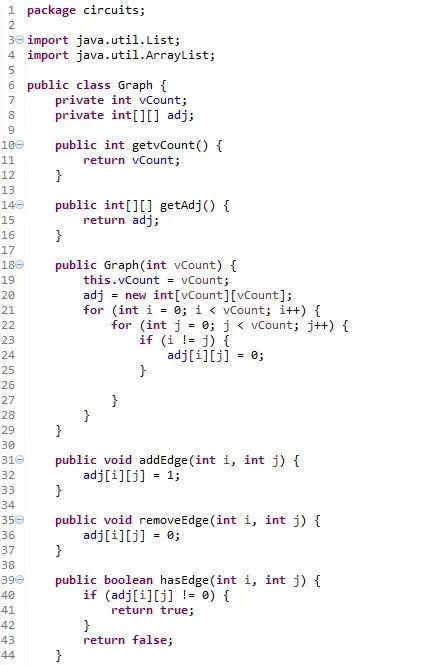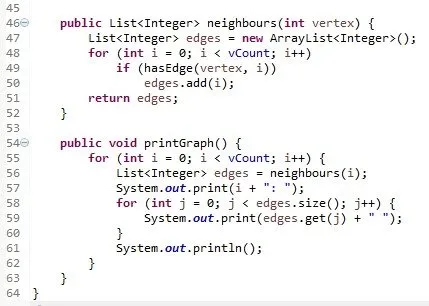The Algorithm consists of the following functions: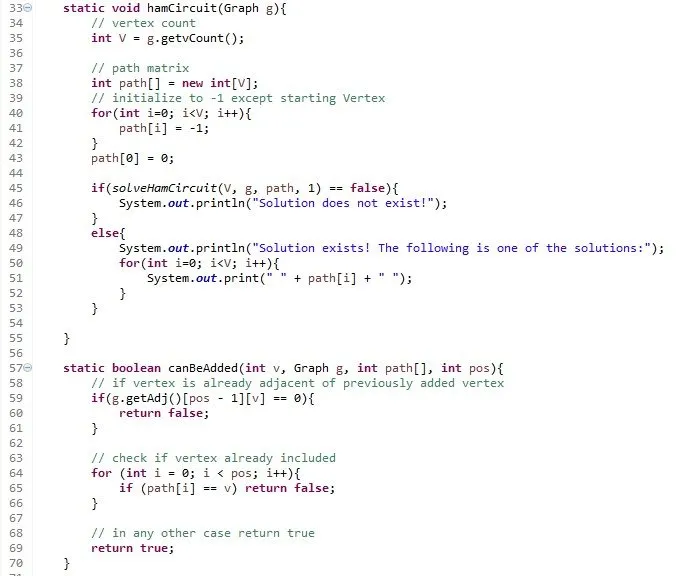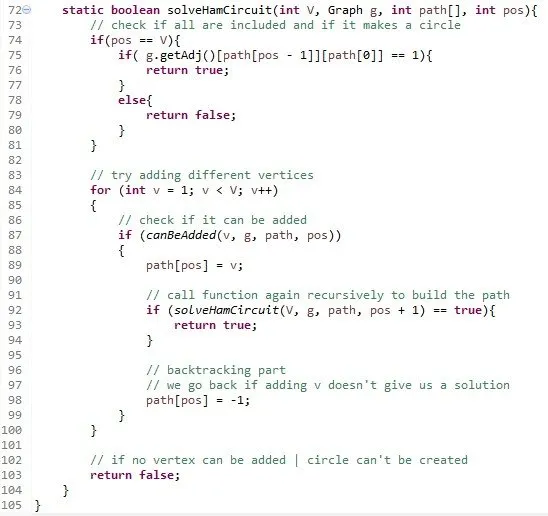I call it inside of this TestGraph class: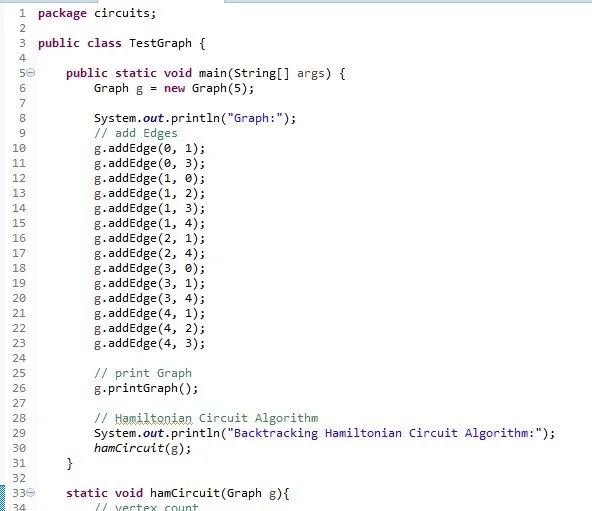Console prints out: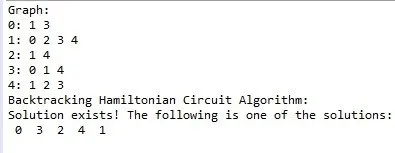For the given Graph we have a Solution!

Try testing it on some other Graphs :P

Graph

TestGraph (Algorithm also)

If you don't want to put it in a package simply delete the declaration...

And this is actually it and I hope that you enjoyed it!

Next time we will implement a Eulerian Circuit Algorithm in Java.

Bye!

H2
H3
H4
3 columns
2 columns
1 column
1 Comment